Home > CC4 > Chapter A > Lesson A.1.9 > ProblemA-98

A-98.

Evaluate the expressions below for the given values. Homework Help ✎

1. 30 − 2x for x = −6

2. x2 + 2x for x = −3

3.x + 9 for x = −6

4.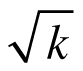for k = 9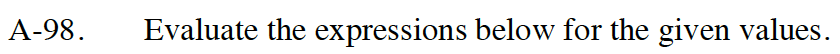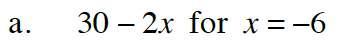Substitute in −6 for x.

30 − 2( −6 )

Evaluate

42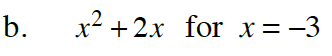Follow the steps in part (a).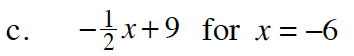Follow the steps in part (a).

12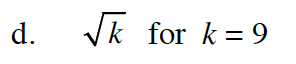Follow the steps in part (a).

3